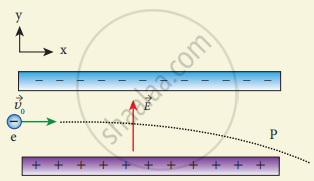Tamil Nadu Board of Secondary EducationHSC Science Class 12th

# Consider an electron travelling with a speed VΦ and entering into a uniform electric field vec"E" which is perpendicular to vec"V"_0 as shown in the Figure. - Physics

Numerical

Consider an electron travelling with a speed VΦ and entering into a uniform electric field vec"E" which is perpendicular to vec"V"_0 as shown in the Figure. Ignoring gravity, obtain the electron’s acceleration, velocity and position as functions of time.#### Solution

Speed of an electron = V0

Uniform electric field = vec"E"

(а) Electron’s acceleration:

Force on electron due to uniform electric field, F = Ee

Downward acceleration of electron due to electric field, a = "F"/"m"

= - "eM"/"M"

Vector from, vec"a" = - "eM"/"M" hat"j"

(b) Electron’s velocity:

Speed of electron in horizontal direction, u = V0 From the equation of motion, V = u + at

V = "V"_0 "eM"/"M" "t"

Vector from vec"V" = "V"_0 hat"j" - "eM"/"M" "t" hat"j"

(c) Electron’s position:

Position of electron, s = r

From equation of motion, r = V0 t + 1/2 (- "eM"/"M")"t"^2

r = V0 t + 1/2 "eM"/"M" "t"^2 hat"j"

Vector from,

vec ""r" = "V"_0 "t" hat "j" 1/2 "eM"/"M" "t"^2 hat"j"

Concept: Electric Dipole and Its Properties
Is there an error in this question or solution?

Share